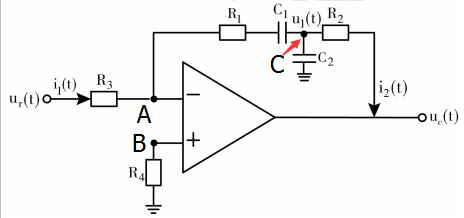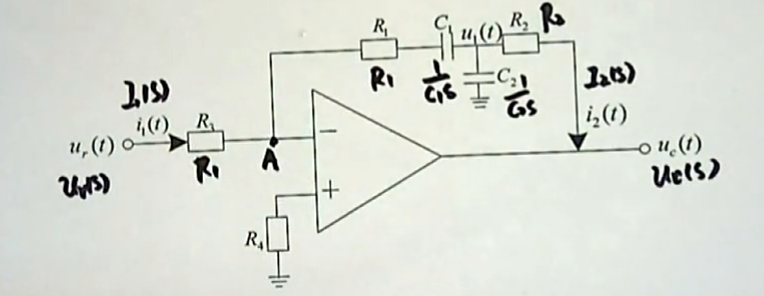# Auto Control 003 自动控制原理 自动控制的数学模型 ---实例讲解

## PID调节器 — 自动控制原理中最简单、最经典、最有用的调节器

1. 写出系统的微分方程；
2. 求系统的传递函数；
3. 说明此电网络在校正中的作用。$$\frac{u{r}(s) - u{A}(s)}{R{3}} = \frac{u{A}(s) - u_1(s)}{R{1} + \frac{1}{C{1}S}}$$

（这样就得到的复频域里面的第一个方程了。）

$$\frac{u{A}(s) - u_1(s)}{R{1} + \frac{1}{C{1}S}} = \frac{u{1}(s) - 0}{\frac{1}{C{2}S}} + \frac{u{1}(s) - u{C}(s)}{R{2}}$$

$$u{A} = u{B} = 0$$